# Coulomb’s Law and Electric Field

0
5525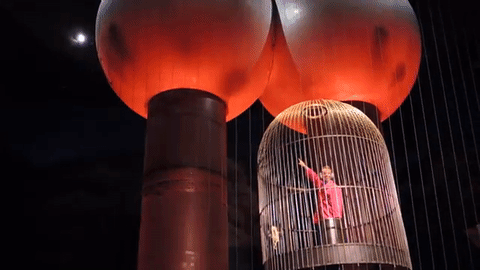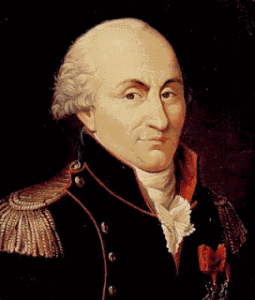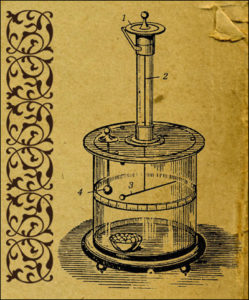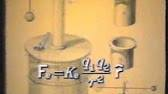Charles-Augustin de Coulomb was a French physicist and inventor. He is known for his contributions in the fields of physics that study electricity and magnetism, and the author of a theory called the Coulomb’s law.
Coulomb defined the electrostatic force (attraction and reflection) using torsion scales, which acts between charged particles. The torque scale consists of a rod connected through the middle of it with thin fibers. The fiber acts as a very weak torsion spring. In Coulomb’s experiment, a torsion scale is an insulating stick with with a metal sleeve at one end attached to a silky net. The ball is charged with known charge for static electricity, and the second charged ball of the same polarity was brought to it. The two charged balls were rejected by one another, twisting the fibers at a certain angle. Knowing how much power it was supposed to spin fibers to a given angle, Coulomb managed to calculate forces between the balls and perform his inverse-squared proportional law.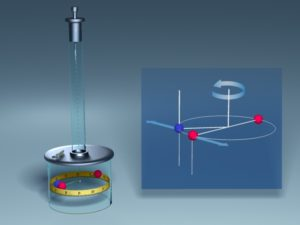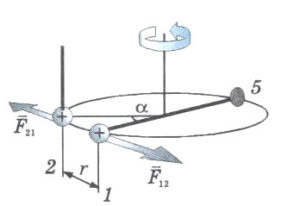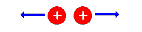### Coulomb’s Law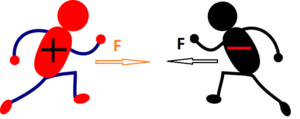The electrical charge of the body or particles is expressed through interaction with other charged bodies. It is known that electric forces are attractive or reflective among electrified bodies, depending on the type of elecricity. Coulomb experimentally established the law of electrostatic interaction of two dotted “charges”, i.e. bodies whose dimensions are much less than the distance between them, or the electrified body of negligible small dimensions is called dotted charge.

Coulomb wanted to confirm the dependence of the electric forces on the distance between the charged bodies and the amount of electricity on these bodies. He came to a law known in physics as the Coulomb’s Law, which we can formulate this way: The strength of the force that attracts or rejects two charges is proportional to their quantities of electricity, and inversely proportional to the square of their mutual distance.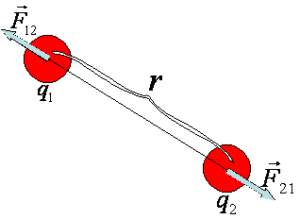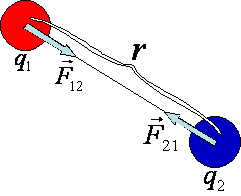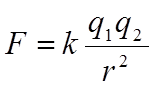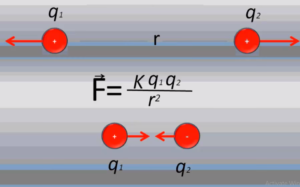The Coulomb force is also called an electrostatic force. The Coulomb force is the vector size, it has the highest value in the vacuum. If several other charged bodies interact with another body, the vector sum of the Coulomb’s forces must be used to determine the resultant force. Expression used for the strength of the electrostatic force when the body is not located in the vacuum is: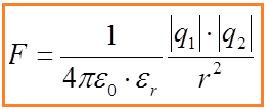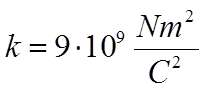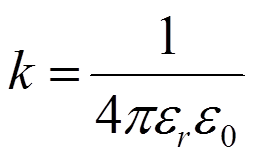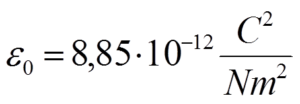The Coulomb force has similarities with gravitational force:
– the strength of both forces decreases with the distance,
– the coulomb force is proportional to the product of the amount of charge
– the gravitational force is proportional to the mass product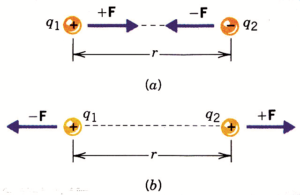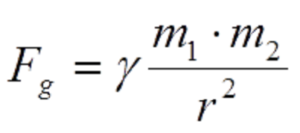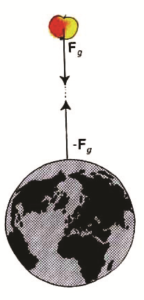In addition, there are differences in the nature of these two forces:
– gravitational force is a very weak force and it acts on a very large scale while Coulomb’s power is stronger and works at small distances
– gravitational force only attracts
– the electrostatic force is both attractive and reflective
– gravitational force does not depend on the environment
– Coulomb’s force depends on the enviroment and has the highest intensity in the vacuum.
– the gravitational force acts between all bodies that have mass
– electric force acts only if the bodies are electrically charged.

### Electric Field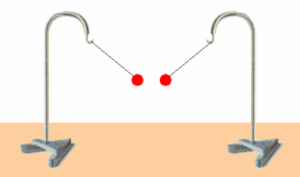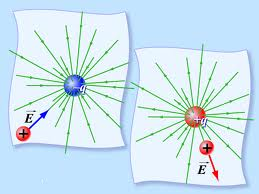The electrified bodies interact through an electric field. The electric field is the transmission of electrical interaction. In the space around the electrically charged body that is stationary, the electrostatic field appears as a special kind of existence of matter.

The charged bodies or particles are usually the “sources” of the electric field. Electric fields reveals its existence by acting with a certain force on the charged bodies found in it. This property determines the physical size, which is called the strength of the electric field.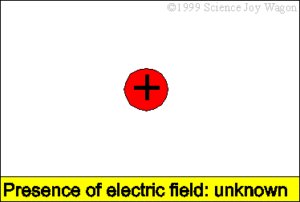F is the force with which the electric field acts on the charge Q, which examines the strength of the electric field.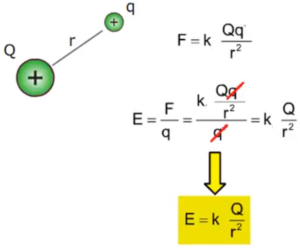Electric field from a point charge :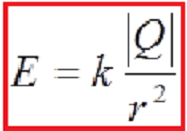The unit for strength of the electric field is 1N/C. The electric field strength is the vector size. The direction of the vector of the electric field strength is the same as the direction of the electrostatic force.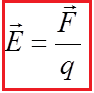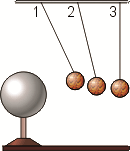Example: Using an electric pendulum, toward the angle of the deflection, it can be concluded that the electric field near the charged body is stronger. As the distance from the body is increasing, it is getting weaker. If the body that creates the field is charged by electrifying the opposite sign, the same pendulum will be removed in the opposite direction, which means that the electric field has a certain strength and direction.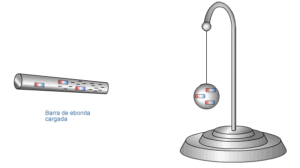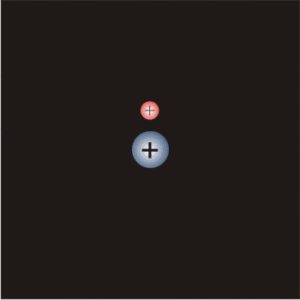Since electric field is a vector quantity, it can be represented by a vector arrow. For any given location, the arrows point in the direction of the electric field and their length is proportional to the strength of the electric field at that location. Such vector arrows are shown in the diagram below.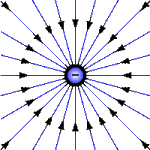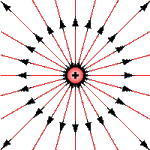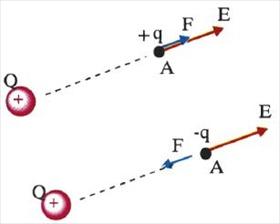Within the electric conductor, the electric field strength is zero, i.e. E = 0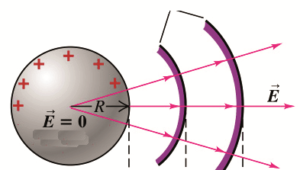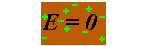Why does the driver feel nothing when the lightning hits the roof of the car? Because there will not be an electric discharge in the car, because the car behaves as a wire ball, and there is no electric field E = 0 inside the conductive shaft.Note that the lengths of the arrows are longer when closer to the source charge and shorter when further from the source charge.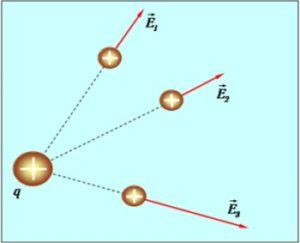We can show the shape of the force line by slipping thin strips of paper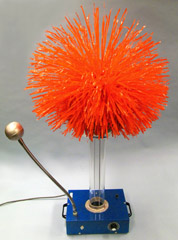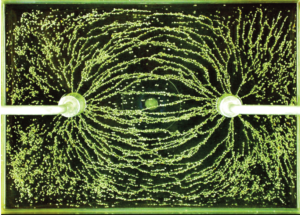Example: The electric field strength lines can be represented by two spheres, one positively and the other negatively charged. We put them under the glass where the castor oil and wheat are sprinkled. The wheat will draw lines as shown below. The figure shows how the electric field strength lines look like between these two charged balls. Animation on the right side shows the lines of the electric field force between the two bodies electrically charged with the opposite sign, while the animation to the left we see the force lines between the postively charged bodies.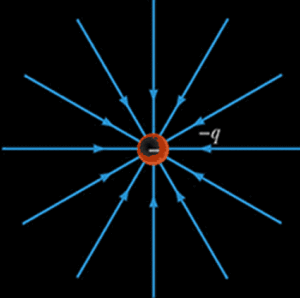The construction of electric field lines in this manner is a tedious and cumbersome task. The use of a field plotting computer software program or a lab procedure produces similar results in less time (and with more phun). Whatever the method used to determine the electric field line patterns for a configuration of charges, the general idea is that the pattern is the resultant of the patterns for the individual charges within the configuration. The electric field line patterns for other charge configurations are shown in the diagrams below.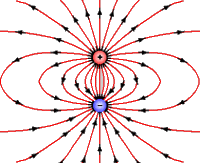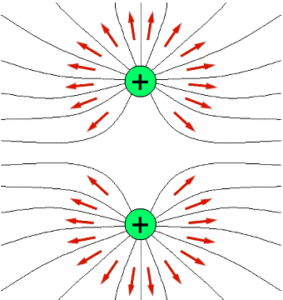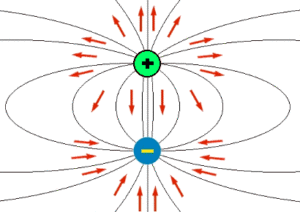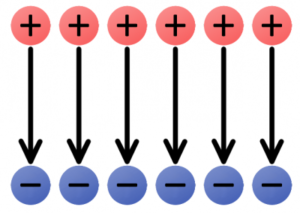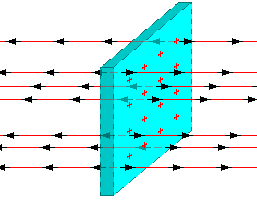If at each point of some part of the space, the electric field has the same magnitude, it is said that there is a homogeneous electric field in that part of the space. With a homogeneous electric field, the force lines are parallel, which shows the animation in the picture below.

SHARE
Previous articleTemperature
Next articleElectric potential energy